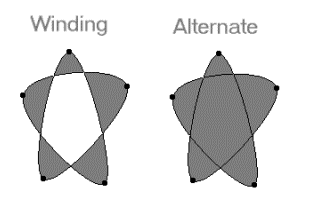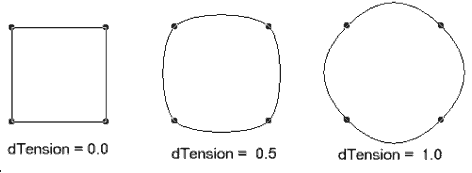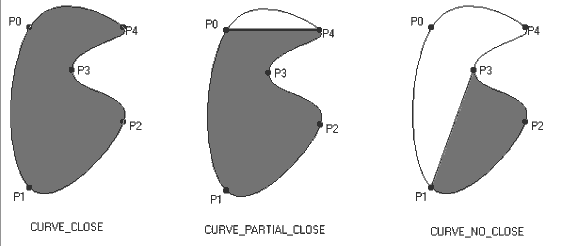﻿ CURVE | Used Structures | Raster Imaging C API Help
In This Topic ▼

# CURVE

````typedef struct tagCurve `
`{ `
`   L_UINT uStructSize; `
`   L_INT nType; `
`   L_INT nPointCount; `
`   L_POINT *pPoints; `
`   L_UINT uFillMode; `
`   L_DOUBLE dTension; `
`   L_INT nClose; `
`   L_INT nReserved; `
`} CURVE, *pCURVE; ````

The CURVE structure defines a curve for the L_SetBitmapRgnCurve function and the L_CurveToBezier function.

## Members

### uStructSize

Size of this structure in bytes, for versioning. Use the sizeof() operator to calculate this value.

### nType

Flag that indicates the type of curve defined. Possible values are:

Value Meaning
CURVE_BEZIER The points specified in the `pPoints` field define a Bezier curve.
CURVE_STANDARD The points specified in the `pPoints` field define a standard curve.
CURVE_NATURAL_CUBIC_SPLINE The points specified in the `pPoints` field define a natural cubic spline curve.

### nPointCount

Number of points in the `pPoints` array.

If `nType` is:

• CURVE_BEZIER, nPointCount must be 3n + 4, where n >= 0. For example, nPointCount can be 4, 7, 10, 13, and so on.

• CURVE_STANDARD, nPointCount must be greater than or equal to 3. For example, nPointCount can be 3, 4, 5, 6, and so on.

• CURVE_NATURAL_CUBIC_SPLINE, nPointCount must be greater than or equal to 3. For example, nPointCount can be 3, 4, 5, 6, and so on.

### pPoints

Pointer to an array of L_POINT structures that contain the points that define the curve.

### uFillMode

Flag that indicates how to handle complex crossing lines. The following are valid values, which are illustrated below:

Value Meaning
L_POLY_WINDING  All pixels that are inside the resulting exterior lines are in the region.
L_POLY_ALTERNATE  The region includes the area between odd-numbered and even-numbered polygon sides on each scan line.### dTension

Value that indicates the "straightness" of the curves that connect points. This value is used only when `nType` is CURVE_STANDARD. Valid values are between 0.0 and 1.0, where 0.0 indicates straight lines, and 1.0 indicates lines with the highest curvature. A typical value for dTension is 0.5.### nClose

Value that indicates how the curve is closed. This value is used only when `nType` is CURVE_STANDARD. Possible values are:

Value Meaning
CURVE_NO_CLOSE [0x0] For n points, draw (n - 3) segments. For example, for points {P0, P1, P2, P3, P4}, draw segments P1P2 and P2P3.
CURVE_CLOSE [0x1] For n points draw (n) segments. For example, for points {P0, P1, P2, P3, P4}, draw segments P0P1, P1P2, P2P3, P3P4, P4P0.
CURVE_PARTIAL_CLOSE [0x2] For n points draw (n - 1) segments. For example, for points {P0, P1, P2, P3, P4}, draw segments P0P1, P1P2, P2P3, P3P4 (but not P4P0).

In the following diagram, the resulting region is shown in gray.### nReserved

Reserved for future use.

The CURVE structure is used by the L_SetBitmapRgnCurve function to draw a curve using an array of POINT items. It is also used by the L_CurveToBezier function to convert a curve to a Bezier. The CURVE structure can define three types of curves:

• `nType` is CURVE_STANDARD: A minimum of three points define a smooth curve that goes through all of the points. In this case, `nPointCount` must be equal to or greater than three.

• `nType` is CURVE_BEZIER: A minimum of four points defines a series of one or more Bezier curves. In this case, `nPointCount` must be 4+3n, where n is equal to or greater than 0. For example, `nPointCount` can be 4, 7, 10, 13, and so on. The first four points define the first Bezier curve, and each subsequent group of three points defines the next Bezier curve, using the last point of the previous Bezier curve as the starting point.

• `nType` is CURVE_NATURAL_CUBIC_SPLINE: A minimum of three points define a smooth curve that imitates the behavior of a plastic rod bent around control pegs (an engineering spline). This behavior can be used to imitate or suggest three-dimensional bending and rotation. In this case, `nPointCount` must be equal to or greater than three.

Some functions which take this structure as a parameter require that the structure be initialized prior to the function call. You must set the `uStructSize` member to the total size, in bytes, of the structure. Use the sizeof() operator to calculate this value. Functions that do not require the structure be initialized will take the total size of the structure, in bytes, as an additional function parameter.

Help Version 21.0.2021.7.5